What is a condensed structure formula?Melody

Solution:

There are different formulas by which a given hydrocarbon can be represented. A given hydrocarbon can be represented by:

1. Molecular formula

2. Structural formula

3. Line formula

4. Condensed formula

5. Lewis formula

Step: 1 Molecular formula is the simplest formula that gives information only about the number of atoms of each element present in the molecule. It does not give any information about the bonding between the atoms.

Step: 1 Representation of a molecule by showing all the atoms and bonding between them with lone pairs is called Lewis formula of the molecule.

Structural formula is similar to Lewis formula except that in structural formula lone pairs on the atoms are not shown.

Step: 3 Line formula is the shorthand notation in which bonds between the carbon atoms and carbon and hydrogen atoms are not shown.

Condensed formula is obtained from the line formula by clubbing together the repeating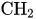units.

The molecular formula of hexane is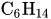.

The line formula is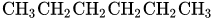.

The condensed formula is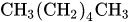.

Similar problems:

Question 1:

Solution:

What is a condensed structure formula for octane?

The molecular formula of octane is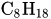.

The line formula is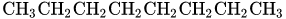.

The condensed formula is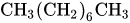.

Question 2:

What is a condensed structure formula for pentane?

The molecular formula of pentane is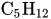.

The line formula is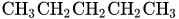.

The condensed formula is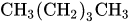.

Need homework help now?

tutors online

Related Questions
2. (11 marks) a) At 400 °C, ammonium iodide decomposes in the following endothermic reaction: NH41(s) + NH3(g) + HI(g) Kp = 0.215 at 400 °C. Write the equilibrium expression for this reaction. 15.0 g of NH4I was placed in an empty 3.0 L flask and heated to 400 °C. What is the partial pressure of NH3 in the flask once the reaction has reached equilibrium? How would the following changes to the equilibrium mixture affect the number of moles of NH3 in the flask and the value of K, once equilibrium is re-established? (Use increase, decrease or stay the same.) Change Effect on moles of NH3 Ke value Increase temperature Increase volume of flask Remove half of HI gas
Notes
Me

OR

Don't have an account?

Join OneClass

Access over 10 million pages of study
documents for 1.3 million courses.

Join to view

OR

By registering, I agree to the Terms and Privacy Policies
Just a few more details

So we can recommend you notes for your school.